# Ratio And Proportion

#### Video Lesson on Ratio And Proportion

A ratio is a comparison of two numbers. We generally separate the two numbers in the ratio with a colon ":". Suppose we want to write the ratio of 8 and 12.

We can write this as 8:12 or as a fraction 8/12, and we say the ratio is eight to twelve.

## Ratio

• The ratio of two quantities a and b of same units is the fraction x/y, where b ? 0
• The fraction x/y can be represented as x:y

## Different types of ratios are:

The concept of ratio & proportion is used in quantitative as well as DI section. Grocery shopping, selling, cooking to industries production- everything makes use of the concept of ratio, proportion & mixtures. Partnership assumes significance in the business world, where various business partners need to distribute profits according to their share of investments and time period. Here, you will learn the formulae & shortcuts to solve questions based on ratio, mixtures and partnership.

### 1) Duplicate ratio:

It is the ratio of squares of two numbers.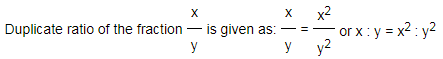### 2) Sub-duplicate ratio:

It is the ratio between square roots of two numbers.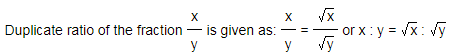### 3) Triplicate ratio:

It is the ratio of cubes of two numbers.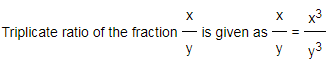### 4) Sub- Triplicate ratio:

It is the ratio between cube roots of two numbers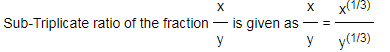### 5) Compound ratio:

It is the ratio of product of first terms in every ratio to that of product of second term in every ratio.
For example: Compound ratio of (a : x), (b : y), (c : z) is (abc : xyz)

### 6) Inverse ratio:

The ratio formed by interchanging their old places in the ratio to new
The inverse ratio of 5 : 8 is 8 : 5.## Proportion:

• 1) Proportion is the equality of two ratios.
When (a : b = x : y) is represented as (a : b :: x : y), then a, b, x, y are said to be in proportion.
In (a : b :: x : y), a and y are called as extremes and b and x are called as mean terms.
Product of means = Product of extremes
• 2) Mean proportion: Mean proportion between x and y is given as xy
• 3) Third proportion: If p : q = q : s, then s is called as third proportional to p and q.
• 4) Fourth proportion: If u : v = x : y, then y is the fourth proportional of u, v and x.

### Quick Tips and Tricks

#### 1) Comparison of ratios:#### 2) Proportion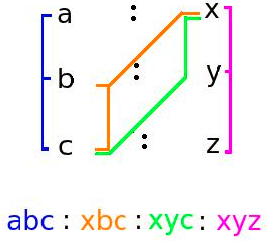#### 4) Variation:

• If a = kb for some constant k, then we can say that a is directly proportional to b.
• If ba =k for some constant k, then we can say that a is inversely proportional to b.

#### 5) Ratio between first and second quantity

If ratio between first and second quantity m : n = a : x, second and third quantity n : p = b : y, fourth and fifth quantity p : q = c : z, then m : n : p : q can be easily solved by using the trick shown below:m : n : p : q = abc : xbc: xyc : xyz

6) If a number a is divided in the ratio x : y,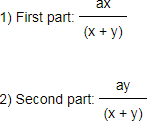### More Educational and Fun StuffMore In-depth knowledge about what you need. Detailed about test preparation, English Writing, TOEFL, and IELTS.